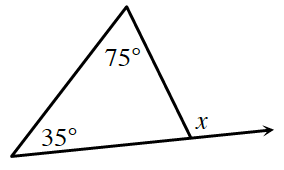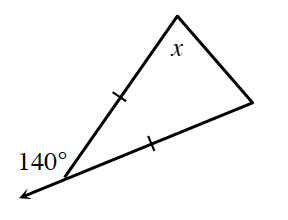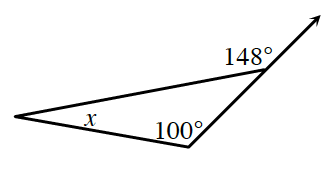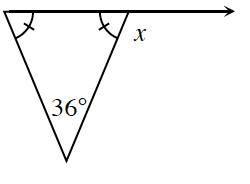### Home > CCG > Chapter 8 > Lesson 8.1.1 > Problem8-6

8-6.

Solve for $x$ in each diagram below.

The Triangle Angle Sum Theorem states that the sum of all the interior angles in a triangle will be $180^\circ$.
Find the missing angle in each triangle.

The sum of supplementary angles is $180^\circ$.
Solve for $x$ using the general equation $\text{missing angle}+x=180^{\circ}$.

1.$x = 110^\circ$

1.$x = 70^\circ$

1.1.